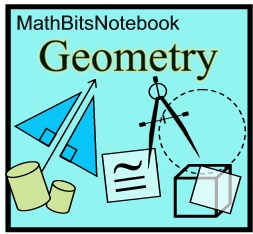Transformations as Functions Terms of Use    Contact Person: Donna Roberts1.
A function machine is shown at the right.
If -3 is input for this function, which choice will be the output?

Choose:
 -11 8 11 13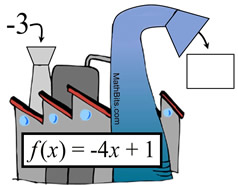2.
A function machine is shown at the right. If the output for this function is -16, which choice was the input value?

Choose:
 -7 7 -4 4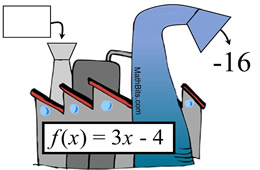3.
A transformation function machine is shown at the right. If point P(2,-5) is input for this function, which choice will be the output (the image)?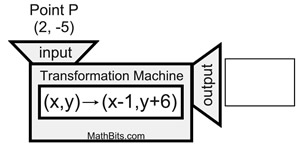Choose:
 P' (3,11) P' (1,1) P' (3,1) P' (1,-1)

4.
A transformation function machine is shown at the right. If image M' (-4,2) is the output from this function, which choice were the coordinates of the pre-image (the input)?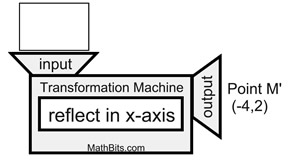Choose:
 M (-4,-2) M (4,2) M (4,-2) M (-4,2)

5.
A translation function is given to be
(x, y) → (x - 5, y + 8).

a) If A(-1,-5), what are the coordinates of A' under this translation?
Choose:
 (6,13) (-6,3) (-4,3) (-4,13)

b)
If B' (6,-2), what are the coordinates of B under this translation?

Choose:
 (1,6) (-1,10) (11,-10) (-11,6)6.
Under a transformation, A (1,6), B (7,-2), and
C (4,-5) become A' (4,5), B' (10,-3), and
C' (7,-6). Which choice is a possible rule for this transformation function?
Choose:
 (x, y) → (x + 3, y - 2) (x, y) → (x - 3, y + 2) (x, y) → (x +3, y - 1) (x, y) → (x - 3, y - 1)7.
The quadrilateral seen at the right will be input into a transformation machine that will rotate the quadrilateral counterclockwise 90º.
a) Which rule will be used by the transformation machine?
Choose:
 (x, y) → (-y, x) (x, y) → (y, x) (x, y) → (-x, -y) (x, y) → (y, -x)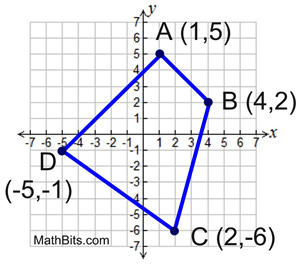b) What will be the coordinates of the output (the image)?
Choose:
 A' (5,-1), B' (2,-4), C' (-6,-2), D' (-1,5) A' (-5,1), B' (-2,4), C' (6,2), D' (1,-5) A' (-1,-5), B' (-4,-2), C' (-2,6), D' (5,1) A' (5,1), B' (2,4), C' (-6,2), D' (-1,-5)

8.
A transformation function takes the line segment from point A(5,2) to point B(-3,4) as input. The function rule (x, y) → (y, x) is applied to the segment.
Which of the choices is the output of the endpoints of the segment?

Choose:
 A(-2,-5); B(-4,3) A(2,-5); B(4,3) A(-2,5); B(-4,-3) A(2,5); B(4,-3)9.
Which of the following transformation function rules will not produce a rigid transformation?

Choose:
 (x, y) → (x + 9, y - 12) (x, y) → (x + 3.5, y + 1.5) (x, y) → (-y, x) (x, y) → (3x, 3y)10.
Which of the following transformation functions produces the same result as a point reflection in the origin?
Choose:
 A reflection in the line y = x. A reflection in the line y = -x. A rotation in the origin of -90º. A rotation in the origin of 180º.